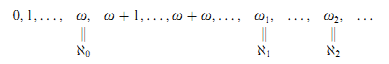Home

Inner Models and Large Cardinals

Extract

In this paper, we sketch the development of two important themes of modern set theory, both of which can be regarded as growing out of work of Kurt Gödel. We begin with a review of some basic concepts and conventions of set theory.

§0. The ordinal numbers were Georg Cantor's deepest contribution to mathematics. After the natural numbers 0, 1, …, n, … comes the first infinite ordinal number ω, followed by ω + 1, ω + 2, …, ω + ω, … and so forth. ω is the first limit ordinal as it is neither 0 nor a successor ordinal. We follow the von Neumann convention, according to which each ordinal number α is identified with the set {ν ∣ ν α} of its predecessors. The ∈ relation on ordinals thus coincides with <. We have 0 = ∅ and α + 1 = α ∪ {α}. According to the usual set-theoretic conventions, ω is identified with the first infinite cardinal ℵ0, similarly for the first uncountable ordinal number ω1 and the first uncountable cardinal number ℵ1, etc. We thus arrive at the following picture:The von Neumann hierarchy divides the class V of all sets into a hierarchy of sets V α indexed by the ordinal numbers. The recursive definition reads:(where} is the power set of x);

V λ = ∪ v Vv for limit ordinals λ. We can represent this hierarchy by the following picture.

References

Hide All
Recommend this journal

Bulletin of Symbolic Logic
• ISSN: 1079-8986
• EISSN: 1943-5894
• URL: /core/journals/bulletin-of-symbolic-logic
Who would you like to send this to? *

×

Metrics

Full text viewsFull text views reflects the number of PDF downloads, PDFs sent to Google Drive, Dropbox and Kindle and HTML full text views.

Total number of HTML views: 0
Total number of PDF views: 0 *Loading metrics...

Abstract viewsAbstract views reflect the number of visits to the article landing page.

Total abstract views: 0 *Loading metrics...

* Views captured on Cambridge Core between <date>. This data will be updated every 24 hours.

Usage data cannot currently be displayed